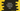# How to implement insertion sort in python## How to implement insertion sort in python:

In insertion sort, list is divided into two parts. One is sorted and another one unsorted. Elements from the unsorted list is picked and placed in the correct position of the sorted list.

Insertion sort is similar to sorting cards in hands. We pick the next card and place it in the correct position of the already sorted cards.

In insertion sort, we pick the first element of the unsorted part and place it in its position in the sorted part.

### Explanation of insertion sort:

Insertion sort works as like below:

• It has two sub lists. One is sorted and another is unsorted.
• The sorted list keeps increasing one by one from the left.
• In the beginning, the sorted part holds the first element of the list and unsorted part holds the rest of the elements.
• In next step, we pick the leftmost element of the unsorted list and put it in the right position of the sorted list.
• Similarly, in the next steps, we keep picking the leftmost element of the unsorted part and keeps putting it in the correct position. Finally, the list will be sorted once the rightmost part become empty.

### Insertion sort in python:

Let’s write it in python:

``````def insertion_sort(arr):
size = len(arr)

for i in range(1, size):
current_value = arr[i]
j = i-1
while j >= 0 and current_value < arr[j]:
arr[j+1] = arr[j]
j -= 1

arr[j+1] = current_value

return arr

if __name__ == '__main__':
arr = [1, 5, 6, 12, 4, 8, 19, 99, 20, 77, 66, 34, 55]
print("Given array : {}".format(arr))
print("Array after insertion sort : {}".format(insertion_sort(arr)))``````

Here,

• insertion_sort method is used to sort a list.
• The for loop iterates from index 1 to the last element of the list.
• For each element, the while loop moves the items and places them at required position.
• Finally the current iterating item is placed at the correct position.

The above program will print:

``````Given array : [1, 5, 6, 12, 4, 8, 19, 99, 20, 77, 66, 34, 55]
Array after insertion sort : [1, 4, 5, 6, 8, 12, 19, 20, 34, 55, 66, 77, 99]``````